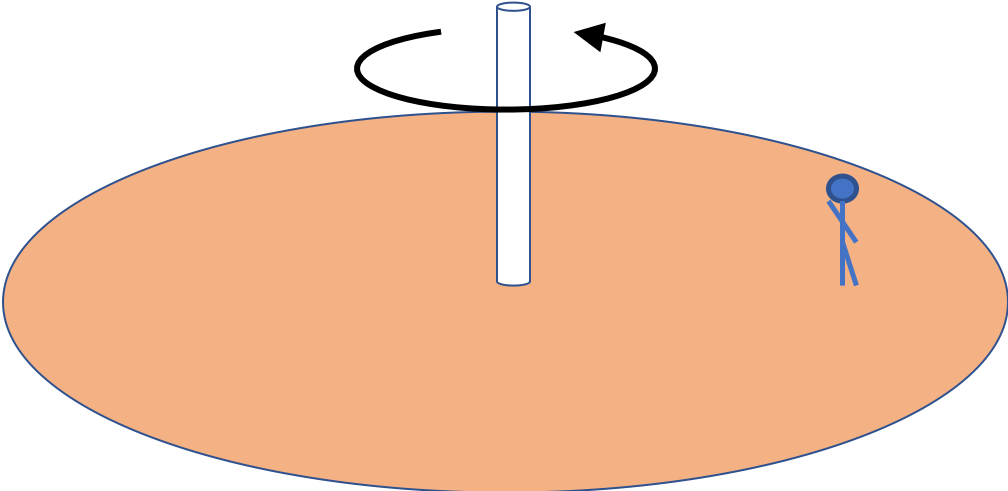## Section9.12Conservation of Angular Momentum

From Newtons's second law for rotational motion, viz., $\mathcal{T}=I\alpha\text{,}$ we have derived above that net rotational impulse equals chave in angular momentum.

\begin{equation*} \vec J_{\text{rot,net,ext}} = \vec L_f - \vec L_i \end{equation*}

From this, we can deduce that if rotational impulse is zero, then angular momentum cannot change.

\begin{equation} \text{If } \vec J_{\text{rot,net,ext}} = 0,\text { then } \vec L_f = \vec L_i.\tag{9.12.1} \end{equation}

This statement is called the principle of the conservation of angular momentum. One simple situation in which $\vec J_{\text{rot,net,ext}}=0$ is when no external torques are acting on a body, e.g., a rotating body in outer space. Such a body will continue to rotate forever at the same rotation velocity. Another situation in which $\vec J_{\text{rot,net,ext}}=0$ is when line of force on the body passes through the axis of rotation, e.g., force by Sun on rotating Earth is approximately like that, and hence, Earth continues to rotate at approximately constant angular speed about an axis towards the North star.

As a concrete example, suppose a spherical rotating star of moment of inertia $I_i$ and angular speed $\omega_i$ suddenly collapses to moment of inertia $I_f$ and ends up rotating at angular speed $\omega_f$ under a force pointed towards the center. Since centrally pointed force will have zero torque, and hence zero rotational impulse, we can say that angular momentum of the star will be cnserved. Therefore, we must have

\begin{equation*} I_i\omega_i = I_f\omega_f. \end{equation*}

An important class of problems where principle of the conservation of angular momentum is very useful is in collisions. In a collision forces are internal and hence $\vec J_{\text{rot,net,ext}}=0\text{.}$ We will see some examples in the next section.

Principles of onservation of momentum, energy, and angular momentum are mire fundamental than Newton's laws of motion and apply to systems where Newton's laws do not hold, e.g. in quantum mechanics.

A disk of mass $M$ and radius $R$ is rotating at an angular speed $\omega$ about an axle through its center and vertical to its plane.

Another disk of mass $M^\prime$ and radius $R^\prime$ falls on the axle and gets stuck as shown in Figure 9.12.2.

The two rotate at a lower speed $\omega^\prime$ about the same axis passing through their common center. Find $\omega^\prime$ in terms of the other quantities given.

Hint

Apply conservation of angular momentum.

$\left( \dfrac{MR^2}{MR^2 + M^\prime {R^\prime}^2}\right)\, \omega\text{.}$

Solution

Since the second disk falls along the direction of the rotating disk, its impulse cannot change the angular momentum around the vertical axis. Due to the sticking of the second disk, the moment of inertia after impact will be the sum of the moments of inertia of the two disks. Let us denote the moments of inertia of the two disks aby $I_1$ and $I_2\text{.}$

\begin{equation*} I_1\, \omega = \left( I_1 + I_2 \right)\, \omega^\prime, \end{equation*}

where

\begin{equation*} I_1 = MR^2,\ \ I_2 = M^\prime {R^\prime}^2. \end{equation*}

Therefore,

\begin{equation*} \omega^\prime = \left( \dfrac{MR^2}{MR^2 + M^\prime {R^\prime}^2}\right)\, \omega. \end{equation*}

A very stunning effect occurs when a neutron star is born. For instsnce, our Sun might one day collapse to a neutron star of $10\text{ km}$ radius neutron star. Currently, the Sun rotates at a spin rate of once every $25\text{ days}$ and has a radius of $7\times 10^{5}\text{ km}\text{.}$ How fast will the Sun be spinning as a neutron star? Give your values in turns per second.

Hint

Use conservation of angular momentum

$227,000$ turns per second.

Solution

Since collapse will invilve only internal forces, angular momentum will be conserved. We assume the star is spherical and use the formula for the moment of inertia of a sphere.

\begin{equation*} \left( \dfrac{2}{5} M R_i^2 \right)\, \omega_i = \left( \dfrac{2}{5} M R_f^2 \right)\, \omega_f. \end{equation*}

Solving for $\omega_f \text{,}$ we get

\begin{equation*} \omega_f = \left( \dfrac{ R_i }{ R_f} \right)^2 \omega_i \end{equation*}

Putting in numbers we get

\begin{equation*} 49\times 10^{10}\times 2\pi\times \dfrac{1}{25} = 1.23\times 10^{11}\text{ rad/day}, \end{equation*}

which is $227,000$ turns per second, which is a bit on the high side. the acutal spin rates are lower than this value due to some loss of angular momentum by exiting particles during the collapse.

Consider a platform of mass $M$ and radius $R$ with a person of mass $m$ standing at a distance $a$ from the center. The platform is initially rotating at angular speed $\omega\text{.}$When the person on the platform walks to another place on the platform with the radial distance $b \lt a\text{,}$ the platform rotates faster.

Find the new rotation speed assuming no change in any external torque being apllied to keep the platform rotating.

Hint

Use conservation of angular momentum on the combined system of person plus the platform.

$\omega_f = \left( \dfrac{\dfrac{1}{2}MR^2 + ma^2 }{\dfrac{1}{2}MR^2 + mb^2} \right)\, \omega.$

Solution

Consider the platform and the person as one system with two parts. When the person moves on the platform, he is exerting force on the platform (and vice-versa) but these forces are all internal for this combined system.

The other objects with which the parts of the system interact are gravity and some motor. The gravity is vertical, which is parallel to the axis, so it does not have torque about this axis. The motor is not putting more torque or reducing its torque. So, we have the condition of no external rotational impulse.

The above arguments justify our use of the conservation of angular momentum. Let $\omega_f$ be the final angular velocity of the platform and the person.

\begin{equation*} \left(\dfrac{1}{2}MR^2 + ma^2 \right)\, \omega = \left(\dfrac{1}{2}MR^2 + mb^2 \right)\, \omega_f. \end{equation*}

Solving this we get

\begin{equation*} \omega_f = \left( \dfrac{\dfrac{1}{2}MR^2 + ma^2 }{\dfrac{1}{2}MR^2 + mb^2} \right)\, \omega. \end{equation*}